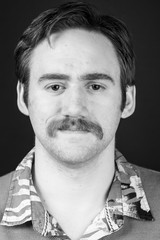# Andries SteenkampFull Name
J.A.J. Steenkamp
Function(s)
Project member
Email
Andries.Steenkamp@cwi.nl
Telephone
+31 20 592 4170
Room
M240
Department(s)
Networks and Optimization

## Biography

Andries Steenkamp is a PhD student of mathematics at Tilburg University under the guidance of Prof. Dr. Monique Laurent. He obtained an MSc. degree at ETHZ in Switzerland in 2018. In 2015 he obtained a BSc. degree in Mathematics from the University of Pretoria in South Africa which was followed with a BSc. Hons. degree in Mathematics in 2016.

## Research

He has a background in inverse problems where he was exposed to generalized moment problems. In particular, the recovery of semi algebraic domains from higher order moments obtained from partial differential equations. Currently his field of study is that of polynomial optimization which has strong links to generalized moment problems. Polynomial optimization is where the objective and constraints are multivariate polynomial functions.  In polynomial optimization he is working on  semidefinite programming based hierarchies for hard to compute matrix factorization ranks. Andries hopes to investigate links between inverse problems, polynomial optimization and approximation hierarchies further.

Current research topics:

• commutative polynomial optimization approach for bounding matrix factorization ranks, and for approximating quantum graph parameters and bounding entanglement dimension in quantum information.
•  convergence analysis of approximation hierarchies.

## Current projects with external funding

• Polynomial Optimization, Efficiency through Moments and Algebra (POEMA)